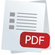# Joule & Watt Meter

Code: IPC-1952-M

Capable of making direct measurement of electrical energy or power supplied to a load and is therefore a valuable tool for pupil based work as well as simplifying many demonstrations (IPC-1952-M). The direct readings mean that the need for voltmeter, ammeter, stop watches and calculators is eliminated and fast and accurate results can be obtained. The science understanding can therefore be gained from scientific investigations rather than mathematical manipulation, making it ideal for pupil based investigations at GCSE, Standard Grade level and A-level in science and technology courses.

Its many uses include experiments with an electrical heater, determination of energy stored in a capacitor, calculating the power supplied by a d.c. source to a resistive load and measurement of the specific latent heat of steam.

The meter is capable of measuring energies from 1mJ to 9999J or power from 1mW to 200W in a.c. or d.c. circuits. Two ranges selected by a switch labelled mJ/mW and J/W are available. The auto-ranging four digit display will give direct readings of energy or power. The maximum current input is 10A. All electrical connections are via 4mm sockets.

Description : Joule & Watt Meter

Part Number : IPC-1952-M

Electrical Supply : 220-240VAC, 50-60Hz

Dimensions : 179 x 190 x 85mm overall

Mass : 1.6kg

Max. input voltage : 20VDC, 14VAC (rms)

Input resistance : 2MΩ (No load

Max. load currents : J/W range = 10A, mJ/mW range = 10mA

Max. energies : J range = 9999J, mJ range = 9999mJ

Max. powers : W range = 200W, mW range = 200mW

Accuracy : Typically ±5% of full scale readingEarn 0 Reward Points

£0.00 £225.79 + VAT